##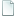Topic: Please help with pathfinding script. (Read 5185 times)previous topic - next topic

0 Members and 1 Guest are viewing this topic.
##### September 26, 2013, 11:15:53 pm
Hello everybody! You may remember me from before the site went down. I came on here pretty regularly. I'm having an issue with my pathfinding script. I can't seem to get it to work. Any chance you could look over the code and find an error? I realize it's not the most efficient but I'm new to pathfinding, so go easy.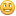Code: [Select]
`var nodeList = [];var pathNodeList = [];var targetX;var targetY;function Node(x, y){	this.x = x;	this.y = y;	this.f = 0;	this.g = 0;	this.h = 0;	this.blocked = false;	this.next;}Node.prototype.copy = function(otherNode){	this.x = otherNode.x;	this.y = otherNode.y;	this.f = otherNode.f;	this.g = otherNode.g;	this.h = otherNode.h;	this.blocked = otherNode.blocked;	this.next = otherNode.next;}function findPath(x1,y1,x2,y2,personName){		var currentX = Math.floor(x1);	var currentY = Math.floor(y1);	targetX = Math.floor(x2);	targetY = Math.floor(y2);	var start = new Node(currentX,currentY);		//Add our initial node to the closed list.	var current = start;		while(true){		nodeList = 		 [null, 			new Node(), null,										new Node(), null, 			new Node(),										null, 			new Node(), null];				findAdjacent(current, personName);				var i = 9;		var f = 2000000000000000000000000000000000000000000000;				var index = 9				while(i--){			if(i % 2 == 0) continue;			if(nodeList[i].blocked == true){				nodeList[i].f = 20000000000;			}			if(nodeList[i].f < f){				f = nodeList[i].f;				index = i;							}					}						nodeList[index].next = current;		var n = new Node();		n.copy(nodeList[index]);		current = n;		if(targetX == current.x){			if(targetY == current.y) break;		}			}			var arr = [];	var nodeListStringDebug = "";		while(current != null){		arr.unshift({x: current.x, y: current.y});		nodeListStringDebug += "  X: " + current.x + " Y:" + current.y		current = current.next;			}	Abort(nodeListStringDebug);}function findAdjacent(parent, nameOfPerson){												nodeList.y =												parent.y - 1;		nodeList.y = 										nodeList.y = 			parent.y;												nodeList.y = 											parent.y + 1;				nodeList.x = parent.x - 1;		nodeList.x = nodeList.x = parent.x;		nodeList.x = parent.x + 1;				var i = 9;				while(i--){						if(i % 2 == 0) continue;						if(IsPersonObstructed(nameOfPerson, nodeList[i].x, nodeList[i].y)) nodeList[i].blocked = true;						if(i % 2 != 0){								nodeList[i].g = parent.g + 10;				nodeList[i].h = 10 * ((nodeList[i].x - targetX) + (nodeList[i].y - targetY)) ^ (1/2);				nodeList[i].f = nodeList[i].g + nodeList[i].h;							}		}}`

• Flying Jester
•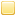• TurboSphere Developer
##### Reply #1 – September 29, 2013, 11:17:30 pm
Out of curiosity, what pathfinding algorithm are you implementing?• N E O
••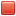##### Reply #2 – September 30, 2013, 02:42:17 pm
It looks like a weighted Dijkstra pathfinder, but at a glance I'm not sure where to even begin searching for code errors.

• Flying Jester
•• TurboSphere Developer
##### Reply #3 – September 30, 2013, 03:53:42 pm
@NEO: Yeah, that got me too.

@devmane144: If you explain the algorithm you are trying to use, it would be a lot easier to help you.

And trust me, pathfinding can be tough. I still can't quite get A* to work just right, and I've been at it for years!##### Reply #4 – September 30, 2013, 11:16:37 pm
It's a really really simplified A* attempt. Get the nodes around the controlled person on each direction. Up down left right. Then use a distance formula to find the h distance, which I originally used a Manhattan Distance formula for, but then shifted to the actual distance formula. Ignore the g value, I only left that in case I were to add diagonal movement eventually. Then try to find the lowest f value and add it to the current node. Basically it stacks nodes, so the first one is inside the second as the "next" variable, the second in the third, etc. Then the pathing code destacks the nodes and returns a list of coordinates. It's not done, I was trying to figure out why it won't find the lowest f value, and it keeps going on forever because it refuses to go the correct direction around obstacles too. Which was the whole point of IsPersonObstructed() being added.

••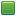• Senior Staff
• Wise Warrior
##### Reply #5 – October 01, 2013, 04:53:56 am
Okay, for one things like this need not be in an A* algo, I'm surprised it works at all!That's a really huge number!

Code: (javascript) [Select]
`var f = 2000000000000000000000000000000000000000000000;`

Second, your code looks confusing so I'll run through my implementation which will hopefully help you out with yours.

Thirdly, your implementation might be very slow. Actually, I don't see separate open and closed lists, which not only speeds things up but makes the backtracking part of it successful.

So, here is my solution.

First create a node object like so:
Code: (javascript) [Select]
`function PathNode(x, y){	this.x = x;	this.y = y;		this.f = 0;	this.g = 0;	this.h = 0;		this.next = null;	this.blocked = false;}`

Notice the 'next' it's because in fact an A* pathing node is a node in a linked list. So not only does it contain data; but a pointer to the next entity in it's list; this will come in handy when linking the correct path.

Next we move on to the initialization step of the algorithm.

Code: (javascript) [Select]
`var Pathfinder = {	open: new BinaryTree(), // binary tree to order opened nodes	closed: [],			    // hash to store closed nodes only			grid: [],				// contains nodes	width: 0,				// width of pathing field	height: 0,				// height of pathing field			init: function(width, height)	{		this.width = width;		this.height = height;				// create the grid here.		var w = 0;		for (var x = 0; x < width; ++x) {			var y = height+1;			while(y--) this.grid[w + y] = new PathNode(x, y);			w += width;		}	}}`

By calling Pathfinder.init(w, h) we would have constructed the field with the width and height of w and h respectively. For a sphere map; you'd use the tile sizes of GetLayerWidth/Height(), but my pathfinder is generalized so you can really use it for any application.

The next step is to run the pathfinder. To do so we make a new method in the global object (which is normally called a 'singleton instance') called "doPath". It looks like the following:

Code: (javascript) [Select]
`	doPath: function(x1, y1, x2, y2) // (x1, y1) is the start; (x2, y2) is the end.	{				this.open.clear();		this.closed = [];		var cur, n = 0, w = 0, h = 0, temp, ww;				temp = this.getStart(x1, y1);		this.closed[temp] = true;		cur = this.grid[temp];		// ...	}`

We clear out our data structures and immediately set the first node to false in the closed list (a list that contains moves that we have taken). Notice the closed list only contains a true or false, it's because we only ever check against this list (it answers the question "have we used this node before?").

Notice I use a method called 'getStart()'. It basically sets up the first node. I often put little code chunks like this into separate functions just to reduce clutter. You want the tightest portion of relevant logic wound into each function call, so as to make most of the space when reading what you are trying to do. A function named 'getStart()' is easily skimmed and we would know what it means, but if not, checking it out isn't that hard. So here is that call:

Code: (javascript) [Select]
`	getStart: function(x, y)	{		var i = x*this.width + y;		var n = this.grid[i];		n.f = n.g = n.h = 0;		return i;	},`

Now, lets continue the "..." portion of the doPath routine.
Code: (javascript) [Select]
`	doPath: function(x1, y1, x2, y2)	{		this.open.clear();		this.closed = [];		var cur, n = 0, w = 0, h = 0, temp, ww;				temp = this.getStart(x1, y1);		this.closed[temp] = true;		cur = this.grid[temp];				while(true) {			w = cur.x + 2;			h = cur.y + 2;						for (var x = cur.x-1; x < w; ++x) {				if (x < 0 || x == this.width) continue;								ww = x * this.width;								for (var y = cur.y-1; y < h; ++y)				{					if (y < 0 || y == this.height) continue;					n = ww + y;										if (this.closed[n]) continue;										temp = this.grid[n];					if (temp.blocked) continue;										if (!this.open.contains(temp)) {						if (x == cur.x || y == cur.y) temp.g = cur.g + 10;						else temp.g = cur.g + 14;						temp.h = 10*(Math.abs(temp.x - x2) + Math.abs(temp.y - y2));						temp.next = cur;						temp.f = temp.g + temp.h;						this.open.add(temp);					}					else if (cur.g < temp.g) {						if (x == cur.x || y == cur.y) temp.g = cur.g + 10;						else temp.g = cur.g + 14;						temp.next = cur;						temp.f = temp.g + temp.h;					}				}			}						if (this.open.root == null) return null;			cur = this.open.shift();			this.closed[(cur.x*this.width)+cur.y] = true;						if (cur.x == x2 && cur.y == y2) break;		}				return this.follow(cur);	},`

So, basically the main body of logic is a double for loop. Each loop does a 3x3 rectangular area of space in the grid and check each neighbor asking the question: 'are you closer to the goal'? At first the node has empty data; so we actually calculate the heuristics right there and then (using Manhattan Distance if I am not mistaken). The 10 and 14 are there to differentiate between diagonal and straight paths. I weighted the diagonals so they are 7 to 5 ratio; which was what was suggested. If the node is indeed closer to our target we move the 'cur' pointer to that new node; adding it to the binary tree and checking it off on the closed list. Whenever such a step is made the next group of reachable nodes is added to the closed list. Of course if the next node we are looking at is blocked we abort early and try the next node thereafter.

And so, the next time we ask for a node we consult the closed list to reduce redundancy, and if it is indeed a new node then it must be in the open list in which case we check a binary tree which has O(log(n)) performance, and quickly find a node that we can use that is closer to the goal. I don't have to use a binary tree; I can use a linear array but it can take awhile to search the values. Once we are finished 'cur' would then point to the node at the position on the end of the path.

The last step is to follow the current pointer back; recreating the path that is most optimum. The method to do that is here.

Code: (javascript) [Select]
`	follow: function(node) {		var arr = [];						while (node) {			arr.unshift({x: node.x, y: node.y});			node = node.next;		}				return arr;	},`

Finally you'll notice that another call is consulted. Quite frankly my system has no way of identifying what is considered blocked or not. Therefore I added an "overrideable" function called block.

Code: (javascript) [Select]
`	block: function(x, y)	{		var i = x*this.width+y;		this.grid[i].blocked = !this.grid[i].blocked;	},`

In this case you call block on all areas (x, y) on the grid that are blocked. If you do not want to do this you can instead check the (x, y) on the map for whether or not the player is blocked on that tile. Again, my pathfinder is setup to be a general one more based on theory and performance than an actual use on top of the map engine; nevertheless it can be easily adapted to fit.

Also dare I say my pathfinder does make quite a bit of use of my binary tree data structure. For one, it does not know how to tell which node is indeed closest. A close node is a node whose 'f' value is the smallest, so I add that logic to my tree for sorting/lookup purposes.

Code: (javascript) [Select]
`Pathfinder.open.compare = function(a, b) {	return a.f > b.f;}`

Full Code (both binary tree and pathfinder)

Uncomment the demo section to see it in action. Make sure pathfinder is the first and only script to run, and that it correctly references the binary tree code (which is itself largely incomplete; only a subset was ever required by the pathfinder).

References
 http://www.policyalmanac.org/games/aStarTutorial.htm
If you use code to help you code you can use less code to code. Also, I have approximate knowledge of many things.

Sphere-sfml here
Sphere Studio editor here

##### Reply #6 – October 01, 2013, 08:47:19 pm
Okay, so being the kind of person I am I tried to recreate this code with my own style, so that I could read it in my own words if you will. I tried the idea of using a linear array instead of your brilliantly designed binary tree, which of course requires a bit more knowledge of binary trees to figure out what you were actually doing there. But binary trees don't make too much sense to me, hence why I decided to use my more familiar arrays, despite the performance cut.

But I reached this point
Code: [Select]
`cur = this.open.shift();this.closed[(cur.x*this.width)+cur.y] = true;`

And I became confused, because the shift function returns the left most branch of the binary tree, which I'm assuming is the lowest in some way shape or form. If I were to translate that into a linear array search, am I looking for the lowest f value from all the nodes in the open list?

• Flying Jester
•• TurboSphere Developer
##### Reply #7 – October 01, 2013, 09:30:52 pm
Shift and unshift are related to push and pop. Shift and unshift return or place the first element, push and pop return or place the last.

You may want to look into array.splice and array.slice.••• Senior Staff
• Wise Warrior
##### Reply #8 – October 01, 2013, 11:07:16 pm

If I were to translate that into a linear array search, am I looking for the lowest f value from all the nodes in the open list?

Yes.
If you use code to help you code you can use less code to code. Also, I have approximate knowledge of many things.

Sphere-sfml here
Sphere Studio editor here

##### Reply #9 – October 03, 2013, 09:18:53 pm
Quote
If I were to translate that into a linear array search, am I looking for the lowest f value from all the nodes in the open list?

Okay let me rephrase that a bit, so that I'm sure what you mean. If I use a linear search algorithm, I am looking for the lowest f value of the nodes created by the 2 for loops around the current point? Or am I searching everything on the open list for the lowest f value? I suppose I could search the whole entire open list as long as I had the current node's f value saved in an initial sort of variable. But that strikes me as kind of inefficient!

••